• 网格简化和 LOD 生成
• 基于外观的联合优化

## 方法总览

### Formulation

• $M_i$ 为原网格 $M$ 的第 $i$ 个简化变体
• $S_i$ 为原 Shader $S$ 的第 $i$ 个简化变体
• $d_i$ 为距相机的距离

$$\epsilon_a (i) = \int_H \| f(M_i, S_i, d_i) - \bar{f}(M, S, d_i) \| dH$$

• $V$ 为离散的若干个 view direction
• $U$ 若干 Shader uniform 参数，如光照方向
• $X \times Y$ 为图像空间的两个维度

$$\epsilon_t (i) = \int_H \| f(M_i, S_i, d_{i+1}) - f(M_{i+1}, S_{i+1}, d_{i+1}) \| dH$$

$$\mathop{\arg \min}_{M_i, S_i, d_i} t = Cost ( f(M_i, S_i, d_i) ) \\ \mathrm{s.t.}\quad \epsilon_a(i) < e_a (d_i) \cdot s_{d_i}$$

$$e_a(d) = (\frac{d-d_{near}}{d_{far} - d_{near}})^Q \cdot e_{max}$$

• $d_{near}$ 和 $d_{far}$ 是设置的视景体参数
• $e_{max}$ 是 maximum absolute per pixel error bound
• 也就是关于 $e_t(i)$ 的积分项关于积分域里面各个部分的最大值
• $Q \in [0, 1]$ 反映了对误差的容忍程度

### 交替优化

•  Y. He, T. Foley, N. Tatarchuk, and K. Fatahalian, “A system for rapid, automatic shader level-of-detail,” ACM Trans. on Graph. (TOG), vol. 34, no. 6, p. 187, 2015.
•  R. Wang, X. Yang, Y. Yuan, W. Chen, K. Bala, and H. Bao, “Automatic shader simplification using surface signal approximation,” ACM Trans. on Graph. (TOG), vol. 33, no. 6, p. 226, 2014.
•  F. Pellacini, “User-configurable automatic shader simplification,”
ACM Trans. Graph., vol. 24, no. 3, pp. 445–452, 2005
•  P. Sitthi-Amorn, N. Modly, W. Weimer, and J. Lawrence, “Genetic programming for shader simplification,” in ACM Transactions on Graphics (TOG), vol. 30, no. 6. ACM, 2011, p. 152.
• Operation Removal: 将 $op(a, b)$ 省略为 $a$ 或 $b$
• Code Transformation: 将 per-pixel 的 pixel shader 操作移动到 per-vertex 或 per-tessellated-vertex 的操作来减少计算量
• Moving to parameter: 将参数用其均值替换（$n \to average(n)$），并且替换到 “parameter stage” 中进行计算（详见 ），并将均值作为结果送入 GPU Shader 中

#### Mesh 简化

Mesh 简化工作：

•  M. Garland and P. S. Heckbert, “Surface simplification using
quadric error metrics,” in Proceedings of the 24th annual conference on
Computer graphics and interactive techniques. ACM Press/AddisonWesley Publishing Co., 1997, pp. 209–216.
•  P. Lindstrom and G. Turk, “Image-driven simplification,” ACM
Transactions on Graphics (ToG), vol. 19, no. 3, pp. 204–241, 2000

QEM

QEM 是 SIGGRAPH’97 提出的经典算法，截至现在已经有大约 5000 次引用。

#### 交替优化

1. 搞 Shader 优化 (然后生成一堆变体 $S_i$)
2. 对于每个在 Pareto frontier 上的 $S_i$，利用该 Shader 进行相应的 Mesh 简化，使得新的 $M_j$ 在满足质量要求 (也就是 error <= absolute error bound) 的情况下为最简

Pareto frontier 上的 $S_i$ 满足

3. 将这些 $(M_j, S_i)$ 按渲染性能排序，取前 20% 作为种子进入下一轮迭代

### 分别优化

#### 生成网格变体

1. First, as has been proven in prior work , the performance and error of shader variants can be predicted instead of being actually evaluated. In this way, we do not need to actually render every shader variant under all scene configurations.

在  中，性能的预测是通过一种简单的启发函数，即 scalar fp ops + 100 * texture ops 来预测的（不同 Shader stage 有不同权重，parameter 数量有额外惩罚）

error 的评价是通过 error cache 和偶尔的重新 evaluate 来实现的

2. Second, we noted that for one shader variant with one simplified mesh, the shading errors at distances could be approximated by filtering the rendered image at the closest distance.

通过在最近距离生成着色结果，再进行 filter 来模拟在远处的结果

3. Finally, we further observed that although these Pareto frontiers may change with scene configurations, the shader variants on Pareto frontiers are similar at similar distances and with similarly simplified meshes.

## 简介

• Section 1: 简介
• Section 2: 解释性的例子
• Section 3: 本文贡献概况
• Section 4: 本文采用的假设和局限性
• Section 5: 收集 kernel 统计信息
• Section 6: 建模 kernel 执行时间
• Section 7: 校准模型参数
• Section 8: 结果展示
• Section 9: 作者调研到的、其它相关的性能建模方法

## 本文的假设和局限性

• (usefulness) 可以帮助用户理解给定机器的性能特性，并且给优化器提供变体性能数据预测参考，同时降低需要在目标系统实际测量的数据数量
• (accuracy) 根据检索到的相关文献显示，在本文提及的 GPU kernel 性能预测问题上，没有方法可以一致的获得小于个位数的预测误差，所以本文也设定这样的目标
• (cost-explanatory): 和其它基于排名的方法不同 (Chen et al. (2018))，虽然本文优化的目标是在各种变体中进行选择，但是本文中模型的主要输出为运行时间，且采用比较可解释的线性模型进行建模

• 硬件资源的利用率：
• 硬件资源的利用率会影响最终的性能。比如，峰值浮点性能受 SIMD lane 使用率影响，片上状态存储器 (VGPR, Scratchpad Memory) 会影响调度槽位的利用率，进而影响延迟隐藏的能力
• 不过，采用本文的方法，基本的性能损失系数是比较容易解释和估计的。比如，实际的内存带宽利用率，以及峰值 FLOP/s
• 即使无法达到硬件资源的全部利用，对于硬件资源利用率随参数变化相对稳定的场合，本文的模型仍然可以适用。不过对于变化的情况，让本文提出的模型适用的唯一可行方法，就是将模型的粒度调低到类似 SIMD lane 的水平，这样利用率的变化就不再相关了。ECM 系列模型就是这样考虑这个问题的。

?

• 为了简化的处理这个问题，本文采用 workgroup size 恒定为 256 的参数设定。
• 程序建模上的简化：
• 本文的模型中，主要检测的是基于某种特殊类别的操作 (e.g. 浮点操作，特殊类型的访存) 和检测到该特征出现的次数，其中次数被建模为 non-data-dependent 的一个特征。
• Polyhedrally-given loop domain?
• 所有分支指令都假设两个分支均会执行，即假设 GPU 采用 masking 的方式进行执行。

• 内存访问开销评估：
• 内存访问的开销受到程序访问的局部性，以及对于 banked memory 来说的 bank 竞争问题的影响。
• 本文将内存访问切分成了两种：
• 对于各个程序都常见的，比较简单的访存模式，用 Section 6.1.1 的办法按 interlane stride, utilization radio 和 data width 进行分类

quasi-affine?

• 对于更复杂的访存模式，在 Section 7.1.1 中提供一种单独抽出来在循环里面按该模式进行访存，并且进行测量的机制
• 平台无关：
• 本文提出的系统作用于 OpenCL 上，但是相似的系统在 CUDA 上也可以比较轻松的实现。

## 收集 kernel 统计信息

### 计数粒度 (count granularity)

• per work-item
• 同步障操作 (barrier synchronization)
• per sub-group （subgroup size 需要用户提供）
• 片上操作：算数指令和 local memory 访问
• uniform 访问：global memory 访问，但是 lid(0) stride 0，即多个线程访问同一块内存区域
• per work-group （没有给出例子）

## 建模 kernel 执行时间

$$T_\text{wall}({\bf n}) = \text{feat}^\text{out}({\bf n}) \approx g(\text{feat}^\text{in}_0({\bf n}), ..., \text{feat}^\text{in}_j({\bf n}), p_0, ..., p_k)$$

• ${\bf n}$ 是整个计算过程中为常数的、仅与各种变体相关的整数向量
• $\text{feat}^\text{in}_j({\bf n})$ 是某种单元特征的出现次数（比如单精度 FP32 乘法数）
• $p_i$ 是硬件相关的校正参数
• $g$ 是用户提供的可微函数

### kernel 特征

#### 数据移动特征

• 内存类别：global / local
• the local and global strides along each thread axis in the array index
• 也就是说，每次 gid(0), gid(1), lid(0), lid(1) 自增一的时候，对 array 数组访问的偏移要分别增加多少
• the ratio of the number of element accesses to the number of elements accessed (access-to-footprint ratio, or AFR)
• AFR = 1: every element in the footprint is accessed one time
• AFR > 1: some elements are accessed more than once
• 这样 Cache 就可能会对速度有加成了

Array Ratio Local strides Global strides Loop stride
a n/16 {0:1, 1:n} {0:0, 1:n*16} 16
b n/16 {0:1, 1:n} {0:16, 1:0} 16*n

With this approach, a universal model for all kernels on all hardware based on kernel-level features like ours could need a prohibitively large number of global memory access features and corresponding measurement kernels. This motivates our decision to allow proxies of “in-situ” memory accesses to be included as features, which in turn motivates our ‘work removal’ code transformation, discussed in Section 7.1.1. This transformation facilitates generation of microbenchmarks exercising memory accesses which match the access patterns found in specific computations by stripping away unrelated portions of the computation in an automated fashion.

Specifying Data Motion Features in the Model: 弄个 aLD, bLD, f_mem_access_tag

#### 算术操作特征

• 操作类型：加法、乘法、指数
• 数据类型：float32, float64

#### 同步特征

• 局部同步障 (local barriers)
• kernel 启动

Recall that the statistics gathering module counts the number of synchronizations encountered by a single work-item, so depending on how a user intends to model execution, they may need to multiply a synchronization feature like local barriers by, e.g., the number of work-groups, a feature discussed in the next section.

A user might incorporate synchronization features into this model as follows:

### 其他特征

• 给定 workgroup count，进行不同 workgroup count 间启动时间补偿
• OpenCL wall time feature
• 给定 platform 和 device 下，执行 60 遍获得平均 walltime，作为输出特征
• “We measure kernel execution time excluding any host-device transfer of data.”

## 校准模型参数

Work Removal Transformation: a code transformation that can extract a set of desired operations from a given computation, while maintaining overall loop structure and sufficient data flow to avoid elimination of further parts of the computation by optimizing compilers

Work Removal 变换会把 on-chip 工作从 kernel 中去掉，达成两方面目的：

1. 测试 on-chip work 和 global memory access 各自占用时间，决定是否要进行 latency hiding
2. 测试某种特殊访存模型的时间占用

### Measurement kernel 设计

• Global memory access
• AFR = 1: Fully specified by local strides, global strides, data size
• That is, patterns that do not produce a write race and not nested inside sequential loops
• Performs global load from each of a variable number of input arrays using the specified access pattern
• Each work-item then stores the sum of the input array values it fetched in a single result array
• Params: data type, global memory array size, work-group dimensions, number of input arrays, thread index strides
• AFR > 1:
• Use Work Removal Tranformation to generate dedicated measurement kernel.
• Arithmetic operations
• First, have each work-item initialize 32 private variables of the specified data type
• Then, perform a loop in which each iteration updates each variable using the target arithmetic operation on values from other variables
• This is to create structural dependency
• We unroll the loop by a factor of 64 and arrange the variable assignment order to achieve high throughput using the approach found in the Scalable HeterOgeneous Computing (SHOC) OpenCL MaxFlops.cpp benchmark (Danalis et al. 2010).
• the 32 variable updates are ordered so that no assignment depends on the most recent four statements
• 32 is used because it permits maximum SIMD lane utilization & prevent from spilling too many registers
• we sum the 32 variable values and store the result in a global array according to a user-specified memory access pattern
• (NOTE: The actual cost can be deduced by change the runcount of arithmetic ops)
• include the global store to avoid being optimized away
• Local memory access
• Tags: data type, global memory array size, iteration count, and workgroup dimensions
• Data type determines the local data stride
1. each workitem initializes one element of a local array to the data type specified
2. Then we have it perform a loop, at each iteration moving a different element from one location in the array to another.
• We avoid write-races and simultaneous reads from a single memory location, and use an lid(0) stride of 1, avoiding bank conflicts.
3. After the loop completes, each work-item writes one value from the shared array to global memory
• Other features
• executes a variable number of local barriers, to measure operation overlapping behaviour (Section 7.4)
• Empty kernel launch, to measure kernel launching overhead

### Operation Overlap 建模

Global memory 和 On-chip 的延迟之间是有可能互相隐藏的。

## 简介

• Program trace
• In software engineering, a trace refers to the record of all states that a program visits during its execution, including all instructions and data.
• 本文提到的 Shader program trace，只包括中间结果 (data)，而不包括程序序列 (instruction)。

Since the fragment shader program operates independently per pixel, we can consider the full program trace as a vector of values computed at each pixel – a generalization from simple RGB.

## 方法

• 输入是用（嵌入到 Python 的） DSL 写的 fragment procedural shader program，翻译成 Tensorflow 程序
• 可以同时输出渲染好的图片和生成的 program trace
• 分支展开、循环 unroll
• These policies permit us to express the trace of any shader as a fixed-length vector of the computed scalar values, regardless of the pixel location

### 输入特征化简

• 编译器优化
• 忽略常量值、计算图上重复的节点，因为其在不同 pixel 位置的运行结果应该高度统一
• 不生成内建函数的 trace
• 检测并筛除迭代改进模式的循环中的中间 trace 结果
• 比如，raymarching 找 closest intersection 的迭代
• 均匀的特征下采样
• The most straightforward strategy is to subsample the vector by some factor n, retaining only every nth trace feature as ordered in a depth first traversal of the compute graph
• 其它采样方案 (都不太好用)
• clustering
• loop subsampling
• first or last
• mean and variance

We first apply compiler optimizations, then subsample the features with a subsampling rate that makes the trace length be most similar to a fixed target length.

For all experiments, we target a length of 200, except where specifically noted such as in the simulation example.

After compiling and executing the shader, we have for every pixel: a vector of dimension N: the number of recorded intermediate values in the trace

### 特征白化

• Check if the distribution merits clamping
• If N <= 10, no need to clamp
• Else, do clamp
• let $P_0$ = Lowest p’th percentile, $P_1$ = highest p’th percentile, superparam $\gamma$
• Clamp to $[P_0 − \gamma(P_1− P_0), P_1 + \gamma(P_1 − P_0)]$
• Do rescale
• for each intermediate feature, rescale the clamped values to the fixed range $[-1,1]$
• Record the bias and scale used (in rescaling)

The scale and bias is recorded and used in both training and testing, but the values will be clamped to range
[-2, 2] to allow data extrapolation.

### 网络

#### 结构

• 1x1 Conv + Feature Reduction (N = 200 -> K = 48)
• 1x1 Conv * 3
• Dilated Convolution (1, 2, 4, 8, 1)
• 1x1 Conv * 3
• 1x1 Conv + Feature Reduction (K = 48 -> 3, that is, RGB color output)

#### 损失函数

$L_b = L_c + \alpha L_p$

GAN related:

## Trace 有效性分析

1. 哪些 Input feature 比较重要？
• 这里作者采用求 Loss 关于 input trace feature 的一阶导数来评价重要性
2. 挑一个 Subset 来做训练？
• 给定 m 个 feature 的训练 budget，如果要评价任意的 subset，即从 N 个里面抽 m 个来做训练的话，开销太大
• Oracle: 按 1 中所述重要性评分的前 m 个 input trace feature
• Opponent: 按 1 中所述重要性评分的后 m 个 input trace feature
• Uniform: 随便挑 m 个
• 发现 Oracle > Opponent > Uniform

## 流程

Update 2023-02-13: 补上了漏掉的创建逻辑设备的一步 vkCreateDevice

• demo_init
• demo_init_connection
• glfwSerErrorCallback
• demo_init_vk
• 启用验证层:
• vkEnumerateInstanceLayerProperties
• demo_check_layers: 检查需要的验证层集合是否存在
• glfwGetRequiredInstanceExtensions: 获得需要的平台 Surface 扩展
• 准备启用的 Instance 扩展列表
• VK_EXT_debug_report
• VK_KHR_portability_enumeration
• vkCreateInstance
• vkEnumeratePhysicalDevices
• 检查设备是否支持 VK_KHR_swapchain
• vkEnumerateDeviceExtensionProperties
• vkCreateDebugReportCallbackEXT
• vkGetPhysicalDeviceProperties
• vkGetPhysicalDeviceQueueFamilyProperties
• vkGetPhysicalDeviceFeatures
• demo_create_window
• glfwWindowHint
• glfwCreateWindow
• glfwSetWindowUserPointer
• glfwSetWindowRefreshCallback
• glfwSetFramebufferSizeCallback
• glfwSetKeyCallback
• demo_init_vk_swapchain
• glfwCreateWindowSurface
• 内部调用 vkCreateWin32SurfaceKHR
• 查找支持 Present 和 Graphics 的 Queue，需要是同一个 Queue
• vkGetPhysicalDeviceSurfaceSupportKHR
• queueFlags & VK_QUEUE_GRAPHICS_BIT
• demo_init_device
• vkCreateDevice: 创建 logical device
• VkDeviceCreateInfo
• .pQueueCreateInfos
• .queueFamilyIndex
• .queueCount
• .pQueuePriorities
• .ppEnabledLayerNames
• .ppEnabledExtensionNames: 要启用的设备扩展

似乎把 Instance 扩展的名字扔进去也行？

• vkGetDeviceQueue
• 选择一个最优的 Surface format
• vkGetPhysicalDeviceSurfaceFormatsKHR
• vkGetPhysicalDeviceMemoryProperties
• demo_prepare
• 创建 Command Pool
• vkCreateCommandPool
• 分配一个 Command Buffer
• vkAllocateCommandBuffers
• VkCommandBufferAllocateInfo:
• .level = VK_COMMAND_BUFFER_LEVEL_PRIMARY
• .commandBufferCount = 1
• demo_prepare_buffers
• 检查 Surface Capabilities 和 Present Modes
• vkGetPhysicalDeviceSurfaceCapabilitiesKHR
• vkGetPhysicalDeviceSurfacePresentModesKHR
• 创建交换链
• 计算 Swapchain Image Extent
• .preTransform 使用 VK_SURFACE_TRANSFORM_IDENTITY_BIT_KHR，如果没有则使用当前 Surface Transform
• .minImageCount 使用 Surface Capabilities 的 minImageCount
• .presentMode 选择 VK_PRESENT_MODE_FIFO_KHR
• vkCreateSwapchainKHR
• 如果有老的交换链： vkDestroySwapchainKHR
• vkGetSwapchainImagesKHR 拿到 VkImage 格式的交换链图像
• 为每个交换链图像调用 vkCreateImageView 创建 Color Attachment View

Componet Swizzle: TODO check spec

• demo_prepare_depth
• vkCreateImage 创建 depth image
• .arrayLayers 可以指定 texture array 的 dimension
• vkGetImageMemoryRequirements 获得 image 的内存要求
• 选择内存大小和内存类型
• memory_type_from_properties : todo check this
• vkAllocateMemory 分配 image 所需内存，返回 VkDeviceMemory
• vkBindImageMemory 将分配的 VkDeviceMemory 绑定到 VkImage
• demo_set_image_layout
• 如果 demo->setup_cmd 为空，则
• 调用 vkAllocateCommandBuffers 从 demo->cmd_pool 中分配 VK_COMMAND_BUFFER_LEVEL_PRIMARY 的 Buffer
• vkBeginCommandBuffer
• 准备 Image Memory Barrier
• VkImageMemoryBarrier
• .srcAccessMask = 0
• 不需要给 src stage 的任何读/写操作 made coherent
• .dstAccessMask:
• 对于 VK_IMAGE_LAYOUT_DEPTH_STENCIL_ATTACHMENT_OPTIMAL，设置为 VK_ACCESS_DEPTH_STENCIL_ATTACHMENT_WRITE_BIT
• .oldLayout = VK_IMAGE_LAYOUT_UNDEFINED，也就是垃圾数据
• .newLayout = VK_IMAGE_LAYOUT_DEPTH_STENCIL_ATTACHMENT_OPTIMAL
• 录制 Pipeline Barrier
• vkCmdPipelineBarrier
• srcStageMask = VK_PIPELINE_STAGE_TOP_OF_PIPE_BIT，也就是 wait for nothing
• dstStageMask = VK_PIPELINE_STAGE_TOP_OF_PIPE_BIT，也就是任何下面的指令在开始前都需要等待 Barrier 执行完
• 同时传入前面的 Image Mmeory Barrier
• vkCreateImageView 创建深度缓冲对应图像的 ImageView
• demo_prepare_textures
• vkGetPhysicalDeviceFormatProperties 获得 VK_FORMAT_B8G8R8A8_UNORM 的 VkFormatProperties
• 对于每张 texture

texture_object 来管理每个 texture

• VkSampler sampler
• VkImage iamge;
• VkImageLayout imageLayout;
• VkDeviceMemory mem;
• VkImageView view;
• int32_t tex_width, tex_height;
• 如果 sampler 支持（对此种 format 的）线性分块 (props.linearTilingFeatures & VK_FORMAT_FEATURE_SAMPLED_IMAGE_BIT)
• demo_prepare_texture_image with required_props = VK_MEMORY_PROPERTY_HOST_VISIBLE_BIT | VK_MEMORY_PROPERTY_HOST_COHERENT_BIT
• vkCreateImage
• vkGetImageMemoryRequirements
• memory_type_from_properties
• 对设备支持的每种内存类型，枚举其是否符合前面 required_props 的要求
• vkAllocateMemory
• vkBindImageMemory
• 如果 memory type 有性质 VK_MEMORY_PROPERTY_HOST_VISIBLE_BIT
• vkGetImageSubresourceLayout
• vkMapMemory: 映射到地址空间
• 填充之
• vkUnmapMemory
• 设置 image layout (前面分析过)
• VK_IMAGE_LAYOUT_PREINITIALIZED -> VK_IMAGE_LAYOUT_SHADER_READ_ONLY_OPTIMAL
• demo_set_image_layout
• 如果 sampler 不支持对此种 format 的线性分块，但支持 optimal 分块 (props.optimalTilingFeatures & VK_FORMAT_FEATURE_SAMPLED_IMAGE_BIT)
• 分别准备 host coherent 和 host visible 的 staging texture 和 GPU device local 的 texture
• demo_prepare_texture_image * 2
• 这里 device local 的显然没能力初始化
• 注意 memory props
• 改 layout 以便使用 transfer 命令
• staging texture: VK_IMAGE_LAYOUT_TRANSFER_SRC_OPTIMAL
• device local texture: VK_IMAGE_LAYOUT_TRANSFER_DST_OPTIMAL
• vkCmdCopyImage
• 将 device local texture 的 layout 改回来
• demo_set_image_layout
• demo_flush_init_cmd: 同步方式 flush setup cmd
• vkEndCommandBuffer
• vkQueueSubmit
• no wait / signal semaphores
• vkQueueWaitIdle
• vkFreeCommandBuffers
• demo->setup_cmd = VK_NULL_HANDLE
• demo_destroy_texture_image 销毁 staging texture
• 创建对应的 sampler 和 Image View
• vkCreateSampler
• vkCreateImageView
• demo_prepare_vertices

这里直接用了 Host visible & Host coherent 的 memory 作为 vertex buffer
而不是 Device local 的，然后单开 staging buffer 做拷贝.

应该是偷懒了.jpg

• vkCreateBuffer
• with .usage = VK_BUFFER_USAGE_VERTEX_BUFFER_BIT
• vkGetBufferMemoryRequirements
• memory_type_from_properties
• vkAllocateMemory
• vkMapMemory
• vkUnmapMemory
• vkBindBufferMemory
• 配置一些结构体
• VkPipelineVertexInputStateCreateInfo
• VkVertexInputBindingDescription
• VkVertexInputAttributeDescription
• demo_prepare_descriptor_layout
• vkCreateDescriptorSetLayout
• VkDescriptorSetLayoutCreateInfo
• .pBindings = &layout_binding
• vkCreatePipelineLayout
• VkPipelineLayoutCreateInfo: demo->pipeline_layout
• 指定了到 Descriptor Set Layouts 的数量和数组指针
• demo_prepare_render_pass
• vkCreateRenderPass
• VkRenderPassCreateInfo
• .pAttachments: VkAttachmentDescription
• : Color Attachment
• .samples = VK_SAMPLE_COUNT_1_BIT 图像的 sample 数
• .loadOp = VK_ATTACHMENT_LOAD_OP_CLEAR color & depth 内容在 subpass 开始时如何处理
• .storeOp = VK_ATTACHMENT_STORE_OP_STORE color & depth 内容在 subpass 结束后如何处理
• .stencilLoadOp = VK_ATTACHMENT_LOAD_OP_DONT_CARE stencil 内容在 subpass 开始时如何处理
• .stencilStoreOp = VK_ATTACHMENT_STORE_OP_DONT_CARE stencil 内容在 subpass 结束时如何处理
• .initialLayout = VK_IMAGE_LAYOUT_COLOR_ATTACHMENT_OPTIMAL subpass 开始前 image subresource 的 layout
• .finalLayout = VK_IMAGE_LAYOUT_COLOR_ATTACHMENT_OPTIMAL subpass 结束后 image subresource 将会被自动转换到的 layout
• : Depth Stencil Attachment
• .format = demo->depth.format
• .samples = VK_SAMPLE_COUNT_1_BIT
• .loadOp = VK_ATTACHMENT_LOAD_OP_CLEAR
• .storeOp = VK_ATTACHMENT_STORE_OP_DONT_CARE
• .stencilLoadOp = VK_ATTACHMENT_LOAD_OP_DONT_CARE
• .stencilStoreOp = VK_ATTACHMENT_STORE_OP_DONT_CARE
• .initialLayout = VK_IMAGE_LAYOUT_DEPTH_STENCIL_ATTACHMENT_OPTIMAL
• .finalLayout = VK_IMAGE_LAYOUT_DEPTH_STENCIL_ATTACHMENT_OPTIMAL
• .pSubpasses: VkSubpassDescription

A single render pass can consist of multiple subpasses. Subpasses are subsequent rendering operations that depend on the contents of framebuffers in previous passes, for example a sequence of post-processing effects that are applied one after another. If you group these rendering operations into one render pass, then Vulkan is able to reorder the operations and conserve memory bandwidth for possibly better performance. Render passes - Vulkan Tutorial

• .pipelineBindPoint = VK_PIPELINE_BIND_POINT_GRAPHICS 该 subpass 支持的 pipeline 类型
• .pInputAttachments = NULL
• .pColorAttachments = &color_reference
• VkAttachmentReference {.attachment = 0, .layout = VK_IMAGE_LAYOUT_COLOR_ATTACHMENT_OPTIMAL}
引用到上面的 
• .pDepthStencilAttachment = &depth_reference
• VkAttachmentReference {.attachment = 1, .layout = VK_IMAGE_LAYOUT_DEPTH_STENCIL_ATTACHMENT_OPTIMAL}
引用到上面的 
• .pDependencies: VkSubpassDependency 有多个 subpass 时指定 subpass 间的读写依赖关系

和 vkCmdPipelineBarrier + VkMemoryBarrier 差不多，区别只是同步作用域限于指定的 subpass 间，而非所有在前在后的操作 (Vulkan Spec)

• demo_prepare_pipeline
• vkCreatePipelineCache: (optional for pipeline creation)

主要用来供实现缓存编译好的 Pipeline; 可以使用 allocator 限制其缓存数据的大小; 可以创建时导入之前 (应用程序) 的 Cache 等

• vkCreateGraphicsPipelines
• VkGraphicsPipelineCreateInfo
• .layout = demo->pipeline_layout
• .pVertexInputState: VkPipelineVertexInputStateCreateInfo
• 已经在 demo_prepare_vertices 中准备好
• .pInputAssemblyState: VkPipelineInputAssemblyStateCreateInfo
• .topology = VK_PRIMITIVE_TOPOLOGY_TRIANGLE_LIST
• .pRasterizationState: VkPipelineRasterizationStateCreateInfo
• .polygonMode = VK_POLYGON_MODE_FILL
• .cullMode = VK_CULL_MODE_BACK_BIT
• .frontFace = VK_FRONT_FACE_CLOCKWISE
• front-facing triangle orientation to be used for culling
• .depthClampEnable = VK_FALSE
• 不启用深度截断
• .rasterizerDiscardEnable = VK_FALSE
• 是否在光栅化阶段前立即丢弃片元
• .depthBiasEnable = VK_FALSE
• .lineWidth = 1.0f
• 光栅化线段宽度
• .pColorBlendState: VkPipelineColorBlendStateCreateInfo
• .pAttachments: VkPipelineColorBlendAttachmentState，对每个 color attachment 定义 blend state
• 
• .colorWriteMask = 0xf
• .blendEnable = VK_FALSE
• 不启用 Blending，直接写入
• .pMultisampleState: VkPipelineMultisampleStateCreateInfo
• .rasterizationSamples = VK_SAMPLE_COUNT_1_BIT
• .pSampleMask = NULL
• .pViewportState: VkPipelineViewportStateCreateInfo
• .viewportCount = 1
• .scissorCount = 1
• 不过这里用的 Dynamic State，也就是 Viewport 和 Scissor 的信息是在录制 Command Buffer 时提供的，创建 Pipeline 时不提供
• 详情看 .pDynamicState
• .pDepthStencilState: VkPipelineDepthStencilStateCreateInfo
• .depthTestEnable = VK_TRUE
• .depthWriteEnable = VK_TRUE
• .depthCompareOp = VK_COMPARE_OP_LESS_OR_EQUAL
• .depthBoundsTestEnable = VK_FALSE
• .stencilTestEnable = VK_FALSE 下面都是 Stencil test 的参数
• .back.failOp = VK_STENCIL_OP_KEEP
• .back.passOp = VK_STENCIL_OP_KEEP
• .back.compareOp = VK_COMPARE_OP_ALWAYS
• .front = ds.back
• .pStages: VkPipelineShaderStageCreateInfo
• 
• .stage = VK_SHADER_STAGE_VERTEX_BIT
• .pName = "main"
• .module = demo_prepare_vs(demo)
• Call demo_prepare_shader_module with vert SPIR-V code
• vkCreateShaderModule with size_t codeSize & uint32_t *pCode
• 
• .stage = VK_SHADER_STAGE_FRAGMENT_BIT
• .pName = "main"
• .module = demo_prepare_fs(demo)
• Similar with above
• .pDynamicState: VkPipelineDynamicStateCreateInfo
• .pDynamicStates = dynamicStateEnables
• 启用了 VK_DYNAMIC_STATE_VIEWPORTVK_DYNAMIC_STATE_SCISSOR
• .renderPass: VkRenderPass
传入之前创建的 VkRenderPass
• vkDestroyPipelineCache
• 删除 vs 和 fs 的两个刚才创建的 Shader Module (demo_prepare_vs / demo_prepare_fs)
• demo_prepare_descriptor_pool
• vkCreateDescriptorPool
• VkDescriptorPoolCreateInfo
• .pPoolSizes = &type_count
• VkDescriptorPoolSize
• .type = VK_DESCRIPTOR_TYPE_COMBINED_IMAGE_SAMPLER
• .descriptorCount = DEMO_TEXTURE_COUNT
• demo_prepare_descriptor_set
• vkAllocateDescriptorSets：按 Descriptor Set Layouts 从 Descriptor Pool 中分配 Descriptor Sets
• .pSetLayouts = &demo->desc_layout
• .descriptorPool = demo->desc_pool
• vkUpdateDescriptorSets
支持 Write 和 Copy 两种形式的 Descriptor Set 更新请求
• VkWriteSescriptorSet
• .dstSet = demo->desc_set 刚分配的 Descriptor Set
• .descriptorCount = DEMO_TEXTURE_COUNT
• .descriptorType = VK_DESCRIPTOR_TYPE_COMBINED_IMAGE_SAMPLER
• .pImageInfo = tex_descs
• VkDescriptorImageInfo: 具体的 Descriptor 内容
• .sampler = demo->textures[i].sampler
• .imageView = demo->textures[i].view
• .imageLayout = VK_IMAGE_LAYOUT_GENERAL

感觉这里应该是选对应的才对，不知道这样可以不可以

• demo_prepare_framebuffers
• 创建 demo->swapchainImageCount 个 VkFramebuffer
• vkCreateFramebuffer
• VkFramebufferCreateInfo
• .renderPass = demo->renderpass
• .pAttachments: VkImageView[]
• : Color Attachment, demo->buffers[i].view
• That is, the swapchain image view
• : Depth Attachment
• demo->depth.view
• .width, .height
• .layers = 1

多 layer 的 Image / Framebuffer 在 Shader 里面是用的 texture array 的语法来访问的

• demo_run
• glfwWindowShouldClose: 检测窗口的 closing 标志
• glfwPollEvent
• demo_draw
• vkCreateSemaphore: imageAcquiredSemaphore
• vkCreateSemaphore: drawCompleteSemaphore
• vkAcquireNextImageKHR

这里有一个问题，这里返回并不意味着 Present 完成 (推荐做法是 Present 设置 Semaphore，然后等 Semaphore)

那么，什么情况下这里会 block？
也可以参考 Let’s get swapchain’s image count straight - StackOverflow

• timeout = UINT64_MAX
• semaphore = imageAcquiredSemaphore
• pImageIndex = &demo->current_buffer: index of the next image to use
• 完成后会 signal 该 semaphore
• 返回值
• VK_ERROR_OUT_OF_DATE_KHR
• demo_resize: 处理 resize 情况：Destroy everything
• vkDestroyFramebuffer
• vkDestroyDescriptorPool
• vkFreeCommandBuffers
• vkDestroyCommandPool
• vkDestroyPipeline
• vkDestroyRenderPass
• vkDestroyPipelineLayout
• vkDestroyDescriptorSetLayout
• vkDestroyBuffer (vertex buffer)
• vkFreeMemory (vertex buffer memory)
• vkDestroyImageView
• vkDestroyImage
• vkDestroySampler
• call demo_prepare
• demo_draw: 重复调用一下自己
• VK_SUBOPTIMAL_KHR: 不是最优，但是也能 present，所以不管
• demo_flush_init_cmd: 同步方式 flush setup cmd
• vkEndCommandBuffer
• vkQueueSubmit
• no wait / signal semaphores
• vkQueueWaitIdle
• vkFreeCommandBuffers
• demo->setup_cmd = VK_NULL_HANDLE
• demo_draw_build_cmd
• vkBeginCommandBuffer: demo->draw_cmd
• vkCmdPipelineBarrier
• Execution barrier 部分
• srcStageMask = VK_PIPELINE_STAGE_ALL_COMMANDS_BIT，也就是 wait for everything
• dstStageMask = VK_PIPELINE_STAGE_BOTTOM_OF_PIPE_BIT (Specifies no stage of execution)

VK_PIPELINE_STAGE_BOTTOM_OF_PIPE_BIT is equivalent to VK_PIPELINE_STAGE_ALL_COMMANDS_BIT with VkAccessFlags set to 0 when specified in the first synchronization scope, but specifies no stage of execution when specified in the second scope.

• Memory barrier 部分: 对 color attachment 做 layout transition
• VK_IMAGE_LAYOUT_UNDEFINED -> VK_IMAGE_LAYOUT_COLOR_ATTACHMENT_OPTIMAL
• .dstAccessMask = VK_ACCESS_COLOR_ATTACHMENT_WRITE_BIT
• vkCmdBeginRenderPass with VK_SUBPASS_CONTENTS_INLINE

VK_SUBPASS_CONTENTS_INLINE specifies that the contents of the subpass will be recorded inline in the primary command buffer, and secondary command buffers must not be executed within the subpass.

• VkRenderPassBeginInfo
• .renderPass
• .framebuffer - 选择当前的 framebuffer，我们有 swapchainImageCount
• .renderArea
• .offset.{x, y}
• .extent.{width, height}
• .pClearValues = clear_values (VkClearValue)

这里是和 RenderPassCreateInfo 指定的 attachments 相对应的

pClearValues is a pointer to an array of clearValueCount VkClearValue structures containing clear values for each attachment, if the attachment uses a loadOp value of VK_ATTACHMENT_LOAD_OP_CLEAR or if the attachment has a depth/stencil format and uses a stencilLoadOp value of VK_ATTACHMENT_LOAD_OP_CLEAR. The array is indexed by attachment number. Only elements corresponding to cleared attachments are used. Other elements of pClearValues are ignored.

•  = {.color.float32 = {0.2f, 0.2f, 0.2f, 0.2f}}
•  = {.depthStencil = {demo->depthStencil, 0}}
• demo->depthStencil 用来加一个“无形的墙”
• vkCmdBindPipeline
• pipelineBindPoint = VK_PIPELINE_BIND_POINT_GRAPHICS
• vkCmdBindDescriptorSets
• layout = demo->pipeline_layout
Recall: Pipeline layout <= Descriptor Set Layouts
• Descriptor Sets
• vkCmdSetViewport
• VkViewport
• .height, .width, .minDepth, .maxDepth
• vkCmdSetScissor
• VkRect2D
• .extent.{width, height}
• .offset.{x, y}
• vkCmdBindVertexBuffers
• firstBinding 参数用于 (CPU 端) 指定绑定到哪里
• vkCmdDraw
• vertexCount = 3
• instanceCount = 1
• firstVertex = 0
• firstInstance = 0
• vkCmdEndRenderPass
• vkCmdPipelineBarrier
• Execution barrier:
• srcStageMask = VK_PIPELINE_STAGE_ALL_COMMANDS_BIT，也就是 wait for everything
• dstStageMask = VK_PIPELINE_STAGE_BOTTOM_OF_PIPE_BIT (Specifies no stage of execution)
• Memory barrier:

正如 transfer，present 也需要 layout 改变

• .srcAccessMask = VK_ACCESS_COLOR_ATTACHMENT_WRITE_BIT
• .dstAccessMask = VK_ACCESS_MEMORY_READ_BIT
• .oldLayout = VK_IMAGE_LAYOUT_COLOR_ATTACHMENT_OPTIMAL
• .newLayout = VK_IMAGE_LAYOUT_PRESENT_SRC_KHR
• vkEndCommandBuffer: demo->draw_cmd
• vkQueueSubmit
• .pCommandBuffers = &demo->draw_cmd
• .pWaitSemaphores = &imageAcquiredSemaphore
• .pWaitDstStageMask = &pipe_stage_flags
• pWaitDstStageMask is a pointer to an array of pipeline stages at which each corresponding semaphore wait will occur.
• 这里设置成了 VK_PIPELINE_STAGE_BOTTOM_OF_PIPE_BIT
• 所以，相当于啥也没等
• .pSignalSemaphores = &drawCompleteSemaphore
• vkQueuePresentKHR
• VkPresentInfoKHR
• .pWaitSemaphores = &drawCompleteSemaphore
• .pSwapchains = &demo->swapchain
• 可以多个，用来支持多个 swapchain 用一个 queue present 操作进行 present
• .pImageIndices = &demo->current_buffer
• 返回值
• VK_ERROR_OUT_OF_DATE_KHR
• demo_resize
• VK_SUBOPTIMAL_KHR
• 啥事不干
• vkQueueWaitIdle
• vkDestroySemaphore: imageAcquiredSemaphore
• vkDestroySemaphore: drawCompleteSemaphore
• demo->depthStencil 周期改变
• vkDeviceWaitIdle
• 如果到了指定的帧数，则 glfwSetWindowShouldClose
• demo_cleanup
• 删除一万个东西 (literally)
• glfwDestroyWindow
• glfwTerminate

TODO: 整理清楚各个维数，因为原论文也不甚详细；

$$L({\bf o}) = \int_{\mathcal{H}^2} L({\bf i}) \rho({\bf i}, {\bf o}) V({\bf i}) \max(0, {\bf n} \cdot {\bf i}) d {\bf i}$$

• ${\bf i}, {\bf o}$ 为入射和出射方向
• $L({\bf i}), L({\bf o})$ 为入射和出射 radiance
• 此处省略了作为参数的 shading point 位置 $\bf x$，下同
• $\rho$ 为 BRDF 函数
• $V$ 为 Visibility 项

$$L({\bf i}) \approx \sum_{i=1}^{n} l_i B_i({\bf i})$$

\begin{aligned} L({\bf o}) &= \int_{\mathcal{H}^2} L({\bf i}) \rho({\bf i}, {\bf o}) V({\bf i}) \max(0, {\bf n} \cdot {\bf i}) d {\bf i} \\ &\approx \sum_i l_i \int_{\mathcal{H}^2} B_i({\bf i}) \rho({\bf i}, {\bf o}) V({\bf i}) \max(0, {\bf n} \cdot {\bf i}) d {\bf i} \\ &= \sum_i l_i T_i({\bf o}) \end{aligned}

$$T_i({\bf o}) \approx \sum_{j=1}^{m} t_{ij} B_j({\bf o})$$

\begin{aligned} L({\bf o}) &\approx \sum_i l_i T_i({\bf o}) \\ &\approx \sum_i l_i \left( \sum_j t_{ij} B_j({\bf o}) \right) \\ &\approx \sum_j \left( \sum_i l_i t_{ij} \right) B_j({\bf o}) \\ \end{aligned}

$$L({\bf o}) \approx \begin{bmatrix} l_1 & ... & l_n \end{bmatrix} \begin{bmatrix} t_{11} & ... & t_{1m} \\ \vdots & & \vdots \\ t_{n1} & ... & t_{nm} \end{bmatrix} \begin{bmatrix} B_1({\bf o}) \\ \vdots \\ B_m({\bf o}) \end{bmatrix}$$

1. 预计算

• 对每个可能的 shading point ${\bf x}$
• 计算该物体的环境光在基函数下对应的系数 $l_i$
• 计算该物体光传输展开系数 $t_{ij}$

当然，对于 Image based lighting，一般认为 $L({\bf i}, {\bf x}) \approx L({\bf i})$，那某些东西就不需要 per-shading point 存储

2. 运行时

• 根据视角 ${\bf o}$ 和位置 ${\bf x}$ 来读取对应的向量并计算

Remarks from paper: PRT methods bake the transport matrix using implicit light sources defined by the illumination basis.
Those light sources shade the asset with positive and negative radiance values. Hence, a dedicated light transport algorithm is used for them.

## 本文思路

• 间接光 $L_i({\bf x}; t)$ 和直接光 $L_d({\bf i}, {\bf x}; t)$ 之间存在线性关系
• 框架：
• 将 ${\bf x}$ 和 $i \times t$ 所在空间分别做一离散化，得到 $I = MD$
• 相当于挑了一组基，每个基内部由同一个光照条件下各个位置的 $L_d$ 组成
• 对于给定的光照条件 $x$ （各个位置 $L_d$的值构成的列向量） ，如何求解 $L_i$ ？
• 首先把 $x$ 分解到该 $D$ 基下，得到系数向量 $c = (D^T D)^{-1} D^T x$
• 每个 $D$ 基我们都存储有对应的输出，所以结果 $y = Mx = I(D^T D)^{-1} D^T x$
• 近似：
• 对 $I$ 进行 SVD 分解并保留前 $k$ 项，得到近似矩阵 $I = U \Sigma V^T \approx U_n \Sigma_n V_n^T$
• $y \approx U_n (\Sigma_n V_n^T) (D^T D)^{-1} D^T x$
• let $M_n = (\Sigma_n V_n^T) (D^T D)^{-1} D^T$
• 存储 $U_n$ 和 $M_n$
• 运行时：
• 用 G-Buffer 得到 $\mathcal{X}_D$ 空间上的各 $L_d({\bf i}, {\bf x}; t)$ 的值
• 计算 $y = U_n M_n x$ 的值

### 估计光传输矩阵

$$L_i({\bf x}; t) = \frac{1}{2 \pi}\int_{\mathcal{H}^2} L_d({\bf i}, {\bf x}; t) V({\bf i}, {\bf x}) \max(0, {\bf n} \cdot {\bf i}) d {\bf i}$$

$L_d({\bf i}, {\bf x}; t)$ 不考虑环境和物体 inter-reflection; 推导中可以先忽略，虽然实际上对于有 inter-transmission 的情况应该也是可以应用的

$$I_k = f(D_k) \quad \forall k \in [0, |\mathcal{T}_D|]$$

$$I = MD$$

$$x = Dc$$

$$Mx = MDc = Ic$$

$$c = (D^T D)^{-1} D^T x$$

$$y = Mx = Ic = I (D^T D)^{-1} D^T x$$

### 间接光基函数

$$I = U \Sigma V^T \approx U_n \Sigma_n V_n^T = U_n C_n$$

\begin{aligned} y &\approx U_n C_n (D^T D)^{-1} D^T x \\ &\approx U_n M_n x \end{aligned}

## 对比经典 PRT

First, because classical PRT restricts the frequency content of the incoming lighting, we can see that the directional light leaks behind the object. Our method does not restrict the frequency content of incoming light but rather the space of possible indirect illumination. Hence, we can better reproduce such lighting scenario.

Furthermore, classical PRT is performed on the vertices of the asset. This can cause interpolation artifacts when the asset is poorly tessellated, and it also links performance to the vertex count. Since we rely on a meshless approach, we are free of issues.

## 局限

Sparse Illumination Measurement. As shown in Section 3.3, the sampling of the measurement points is linked to the achievable lighting dimensionality. Thus, it needs to be sufficiently dense to reproduce the space of observable lighting configurations. It follows that a lighting scenario mixing many light types might require a denser sampling.

No Directionality. We reconstruct a diffuse appearance when reconstructing indirect illumination. However, since our method does not depend on the encoding of the measured indirect illumination, it can be extended to reconstruct glossy appearances e.g. directional distributions using directional sampling or any basis such as Spherical Harmonics. However, our method is likely to be restricted to low frequency gloss here and will not work to render specular reflections.

Large Assets. Our solution is not designed to handle assets such as levels in a game. Because we handle light transport globally and reduce it with a handful of basis functions, we cannot reconstruct the interconnected interiors or large environments in which the combinatorics of possible illumination is large. For such case, our method would require to be extended to handle modular transfer between disjoint transport solutions (Similar to Loos et al. ).

## 多重重要性采样 (MIS)

for Monte Carlo Rendering

$$I = \int_\Omega f(x) dx$$

$$\langle I \rangle_{mis} = \sum_{i=1}^m \frac{1}{n_i} \sum_{j=1}^{n_i} \frac{w_i(x_{i,j}) f(x_{i, j})}{p_i(x_{i,j})}$$

\begin{aligned} \operatorname{E}[\langle I \rangle_{mis}] &= \operatorname{E}\left[ \sum_{i=1}^m \frac{1}{n_i} \sum_{j=1}^{n_i} \frac{w_i(x_{i,j}) f(x_{i, j})}{p_i(x_{i,j})} \right] \\ &= \sum_{i=1}^m \frac{1}{n_i} \operatorname{E}\left[ \sum_{j=1}^{n_i} \frac{w_i(x_{i,j}) f(x_{i, j})}{p_i(x_{i,j})} \right] \\ &= \sum_{i=1}^m \operatorname{E}\left[ \frac{w_i(x_{i,1}) f(x_{i, 1})}{p_i(x_{i,1})} \right] \quad (\because \text{i.i.d})\\ &= \sum_{i=1}^m \int_\Omega \frac{w_i(x) f(x)}{p_i(x)} p_i(x) dx \\ &= \sum_{i=1}^m \int_\Omega w_i(x) f(x) dx \\ &= \int_\Omega \sum_{i=1}^m w_i(x) f(x) dx \\ &= \int_\Omega f(x) dx \\ &= I \end{aligned}

$$\hat w_i (x) = \frac{c_i p_i(x)}{\sum_{j=1}^m c_j p_j(x)} \quad \text{where}\ c_i = n_i / \sum_{j=1}^{m} n_j$$

$$\operatorname{V}[\langle \hat I \rangle_{mis}] \le \operatorname{V}[\langle I \rangle_{mis}] + \left( \frac{1}{\min_i n_i} - \frac{1}{\sum_i n_i} \right) I^2$$

$$\langle I \rangle_{mis} = \frac{w_t(x_{t,1}) f(x_{t,1})}{p_t(x_{t,1}) P(t=i)}$$

Veach 的论文中证明，Balance Heuristic 在任何 One-sample MIS 的情形下都是最优的权重组合。

## 连续多重重要性采样 (Continuous MIS)

West 等人将上面的工作进行了进一步的推广：如果现在有连续的无限多种采样策略，那么也可以将 MIS 中的估计量进行推广，得到连续多重重要性采样 (Continuous Multiple Importance Sampling, CMIS)。

$$\langle I \rangle_{CMIS} = \frac{w(t, x)f(x)}{p(t, x)} = \frac{w(t, x)f(x)}{p(t) p(x|t)}$$

1. $\int_\mathcal{T} w(t, x) dt = 1$ 对任何 $x \in \operatorname{supp} f(x)$ 成立
2. 当 $p(t, x) = 0$ 时，$w(t, x) = 0$

为什么？

$$\bar w(t, x) = \frac{p(t)p(x|t)}{\int_\mathcal{T} p(t') p(x|t') dt'} = \frac{p(t, x)}{\int_\mathcal{T} p(t', x)dt} = \frac{p(t, x)}{p(x)}$$

## 随机多重重要性采样 (Stochastic MIS)

SMIS 首先假设在 $\mathcal{T} \times \mathcal{X}$ 中独立的采样 $(t_1, x_1), …, (t_n, x_n)$ 共 $n$ 组点。

TODO: implement me

### 应用

#### Volume Single Scattering

DXGI (DirectX Graphics Infrastructure) 负责抽象和交换链、DAL 相关的公共部分。关于 DXGI 的资料可以参考 MSDN

DXGI 封装了多种对象：

• 显示适配器 (IDXGIAdapter): 一般对应一块显卡，也可以对应 Reference Rasterizer，或者支持虚拟化的显卡的一个 VF 等
• 显示输出 (IDXGIOutput): 显示适配器的输出，一般对应一个显示器
• 交换链 (IDXGISwapChain): 用来暂存要显示到输出窗口/全屏幕的 1 到多个 Surface 的对象
• g_pSwapChain->GetBuffer 可以拿到表示 Back Buffer 的 ID3D11Texture
• g_pd3dDevice->CreateRenderTargetView 来创建一个封装该 Texture 的 ID3D11RenderTargetView
• g_pd3dDeviceContext->OMSetRenderTargets 来设置管线的 RenderTarget
• g_pd3dDeviceContext->RSSetViewports 来设置管线的 Viewport

## GameApp::Init()

• D3DApp::Init()
• InitMainWindow()
• RegisterClass(WNDCLASS *): 注册
• AdjustWindowRect()
• CreateWindow()
• ShowWindow()
• UpdateWindow()
• InitDirect3D()
• D3D11CreateDevice(): 采用 11.1 的 Feature Level，不行则降级

该函数会返回 Immediate Context (ID3D11DeviceContext), 设备 (ID3D11Device) 和特性等级

• ID3D11Device::CheckMultisampleQualityLevels: 查询给定 DXGI_FORMAT 是否支持给定倍数的 MSAA

• 将前面的 ID3D11Device Cast 到 IDXGIDevice

An IDXGIDevice interface implements a derived class for DXGI objects that produce image data.

• IDXGIDevice::GetAdapter 拿到 IDXGIAdapter

The IDXGIAdapter interface represents a display subsystem (including one or more GPUs, DACs and video memory).

• IDXGIAdapter::GetParent 拿到 IDXGIFactory1

这里的 GetParentIDXGIAdapter 作为 IDXGIObject 的方法，可以获得构造它的工厂类。

The IDXGIFactory1 interface implements methods for generating DXGI objects.

• 尝试将 IDXGIFactory1 Cast 到 IDXGIFactory2 (DXGI 1.2 新增)

• 如果支持 DXGI 1.2，则用 CreateSwapChainForHwnd 来创建交换链
• 否则，用 CreateSwapChain 来创建交换链

这两个函数都可以创建窗口 / 全屏幕交换链；DXGI 1.2 增加了新的、到其它输出目标的交换链创建功能，所以这里进行了重构。

也要注意，不同 DirectX 可以支持的交换链的交换行为类型是不同的。大体上，交换链的交换行为可以分为

• DISCARD vs SEQUENTIAL: 可以参考 StackExchange，区别就是一个驱动可以放心扔掉，另一个必须保留回读可能
• FILP vs BLIT (Bit Block Transfer): 决定是用交换指针还是数据拷贝的方法来从交换链被 Present 的 Surface 中拿取数据
• IDXGIFactory1::MakeWindowAssociation 来取消让 DXGI 接收 Alt-Enter 的键盘消息并且切换窗口和全屏模式

• D3D11SetDebugObjectName()

• ID3D11DeviceChild::SetPrivateData(WKPDID_D3DDebugObjectName, ...) 来设置资源的内部数据，这里是调试名称
• DXGISetDebugObjectName()

• IDXGIObject::SetPrivateData(WKPDID_D3DDebugObjectName, ...) 来设置资源的内部数据，这里是调试名称
• OnResize()

• IDXGISwapChain::ResizeBuffers()
• IDXGISwapChain::GetBuffer() 拿到 ID3D11Texture2D 形式的 Back Buffer
• IDXGISwapChain::CreateRenderTargetView() 创建绑定到上面 Back Buffer 的 Texture 的渲染目标视图
• D3D11SetDebugObjectName 来设置 Back Buffer 的调试名称
• ID3D11Device::CreateTexture2D() 来创建深度模板缓冲 (Depth Stencil Buffer)，类型 ID3D11Texture2D，包含大小，MipLevel，采样描述等
• ID3D11Device::CreateDepthStencilView() 来创建前面缓冲对应的深度模板视图
• ID3D11DeviceContext::OMSetRenderTargets() 来将渲染目标视图和深度木板视图绑定到管线
• ID3D11DeviceConetxt::RSSetViewports() 绑定 Viewport 信息到光栅器状态
• D3D11SetDebugObjectName() 设置调试前面各种视图对象的对象名
• InitEffect()
• CreateShaderFromFile(): 传入 CSO (Compiled Shader Object) 和 Shader 文件，输出 ID3DBlob *
• 如果有缓存，则用 D3DReadFileToBlob 装入，并返回
• D3DCompileFromFile(): 编译并生成 ID3DBlob 对象
• 如果指定了缓存路径，则 D3DWriteBlobToFile() 进行输出

分别创建了 vs_5_0ps_5_0 Shader Model 的 Shader Blob

• ID3D11Device::CreateVertexShader()，根据 Shader Bytecode 创建 ID3D11VertexShader 对象

TODO: 研究一下

• ID3D11Device::CreateInputLayout() 传入输入元素描述符和 Shader，传出 ID3D11InputLayout 对象
• ID3D11Device::CreatePixelShader() ，根据 Shader Bytecode 创建 ID3D11PixelShader 对象
• InitResource()
• ID3D11Device::CreateBuffer() 创建顶点缓冲区 (ID3D11Buffer) ，并传入初始化数据
• ID3D11Device::CreateBuffer() 创建索引缓冲区 (ID3D11Buffer) ，并传入初始化数据
• ID3D11DeviceContext::IASetIndexBuffer() 设置 Immediate Context 绑定索引缓冲区
• ID3D11Device::CreateBuffer() 创建常量缓冲区 (ID3D11Buffer) ，不是用初始化数据
• 此处设置 D3D11_BUFFER_DESCCPUAccessFlagsD3D11_CPU_ACCESS_WRITE，让 CPU 可以改变其值
• ID3D11DeviceContext::IASetVertexBuffers() 设置顶点缓冲区，stride 和 offset
• ID3D11DeviceContext::IASetPrimitiveTopology() 设置图元类型
• ID3D11DeviceContext::IASetInputLayout() 设置输入布局
• ID3D11DeviceContext::VSSetShader() 绑定顶点着色器到管线
• ID3D11DeviceContext::VSSetConstantBuffers() 设置常量缓冲区

这里当然是拿着 ID3D11Buffer 去设置

• ID3D11DeviceContext::PSSetShader() 设置像素着色器
• D3D11SetDebugObjectName() 将 Input Layout, Shader 和 Buffer 设置好调试用名字

## GameApp::Run()

• WM_SIZE: 如果在 WM_ENTERSIZEMOVEWM_EXITSIZEMOVE 中间，则忽略，否则调用 OnResize() 重新配置交换链并绑定到管线
• WM_ACTIVATE: 窗口不活跃时暂停渲染
• WM_DESTROY: 窗口退出消息

• CalculateFrameStats(): 根据定时器计算时长并更新窗口标题
• UpdateScene(): 更新场景 (主要是更新常量缓冲)
• 计算更新后的常量缓冲区值
• ID3D11DeviceContext::Map 传入 Constant Buffer 对象

MSDN: Gets a pointer to the data contained in a subresource, and denies the GPU access to that subresource.

这里要指定映射类型 (CPU 可读，CPU 可写，CPU 可写且原内容可放弃)；不过，这里还有一种类型，叫做 D3D11_MAP_WRITE_NO_OVERWRITE，这块 MSDN 的文档有比较详细的解释。

也可以看看这篇知乎专栏作为参考。

• memcpy(mappedData.pData, &cpuCBuffer, sizeof(cpuConstBuffer)) 将数据拷贝到 D3D11_MAPPED_SUBRESOURCE::pData 成员处
• ID3D11DeviceContext::Unmap 解除内存映射
• DrawScene(): 绘制场景
• ID3D11DeviceContext::ClearRenderTargetView(): 用给定颜色清空渲染目标视图
• ID3D11DeviceContext::ClearDepthStencilView(): 用给定深度和模板值清空深度模板视图
• ID3D11DeviceContext::DrawIndexed(): 绘制给定的立方体
• IDXGISwapChain::Present(SyncInterval=0, flags=0): 告知交换链已经完成绘制，可以呈现，并且要求立即呈现

IDXGISwapChain::Present: Presents a rendered image to the user.

## NeRF 回顾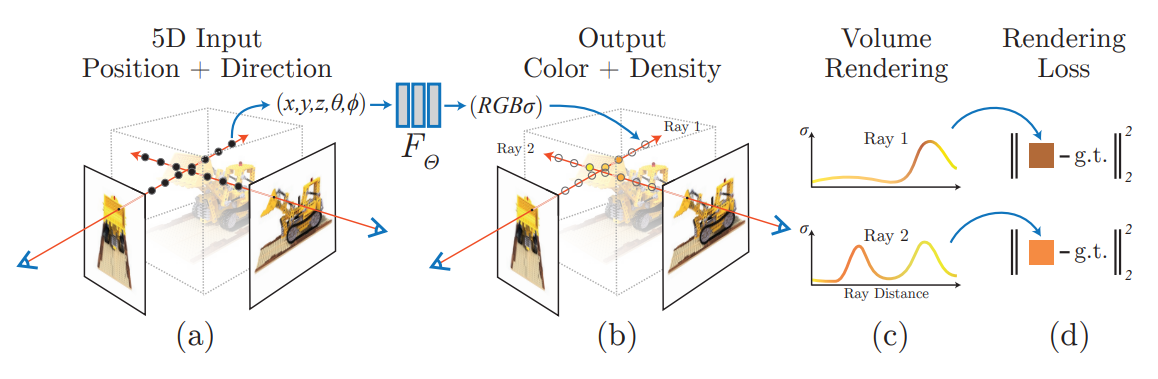Neural Radiance Field，简称 NeRF，最开始在 ECCV 2020 上被提出，提出了以神经网络编码辐射场的一种技术，并且将其运用到了基于图片的场景重建等多个领域中，是近年来受关注度相当高的一篇工作。

NeRF 的网络部分输入为 5D: 位置 $(x,y,z)$ 和朝向 $(\theta, \phi)$，输出为该位置的 RGB 颜色和密度。

NeRF 在给定相机位置下最终渲染的输出用类似体渲染 (Volumetric Rendering) 的办法来实现。

### NeRF 体渲染

$$C({\bf r}) = \int_{t_n}^{t_f} T(t) \sigma({\bf r}(t)) {\bf c}({\bf r}(t), {\bf d}) dt$$

• $T(t) = \exp (-\int_{t_n}^{t} \sigma({\bf r}(s)) ds )$ 为光线从 $t_n$ 能打到 $t$ 的概率
• 比如说，如果射线穿过的部分密度都比较大，那 $T(t)$ 就会比较小
• $\sigma({\bf r}(t))$ 是该 $t$ 对应的点 ${\bf r}(t)$ 的密度
• ${\bf c}({\bf r}(t), {\bf d})$ 是网络给定方向和位置后输出的 RGB 颜色值
• $t_n$ 和 $t_f$ 分别为射线进入和射出 NeRF 有效区域的包围盒时所对应的最近和最远参数值

$$\hat C({\bf r}) = \sum_{i=1}^{N} T_i (1-\exp(-\sigma_i \delta_i)) {\bf c}_i$$

• $T_i = \exp(- \sum_{j=1}^{i-1} \sigma_j \delta_j)$
• $\delta_i = t_{i+1} - t_i$ 为两临近采样点的距离

### NeRF 网络

#### 位置编码

$$\gamma(p) = (\sin(2^0 \pi p), \cos(2^0 \pi p), ..., \sin(2^{L-1} \pi p), \cos(2^{L-1} \pi p))$$

#### Coarse & Fine MLP

NeRF 同时使用两个 MLP 来表示场景，一个粗粒度 MLP 和一个细粒度 MLP。

$$\hat C_c(r) = \sum_{i=1}^{N_c} w_i c_i, \quad w_i = T_i (1-\exp(\sigma_i \delta_i))$$

## ICARUS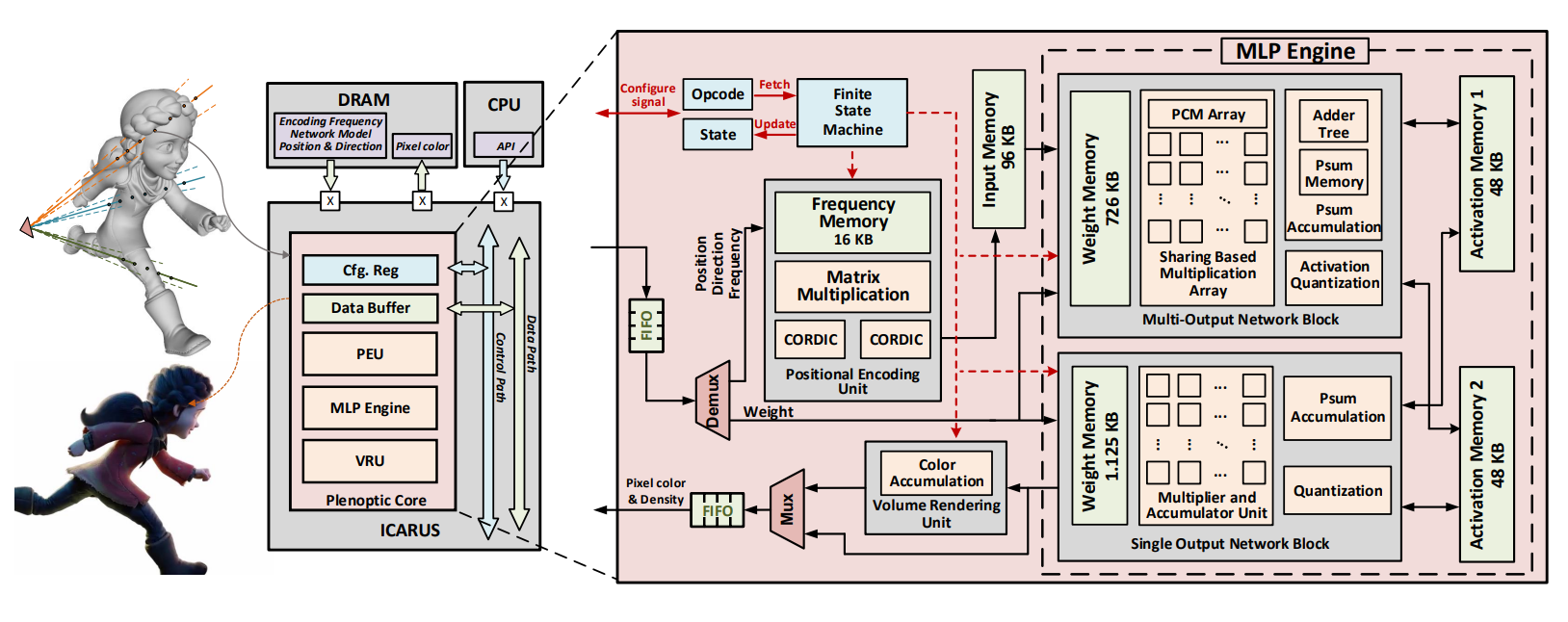### NeRF 计算过程回顾

1. 对像素所发出射线上的采样，得到点 $({\bf p}_1, …, {\bf p}_N)$
2. 查询 MLP 网络：$({\bf p}_i, {\bf d}_i) \to ({\bf c_i}, \sigma_i)$
3. 进行多次 alpha-blending

### 架构设计

1. “端到端” - 芯片输入位置和方向，输出像素颜色，减少片上片外数据交换的额外开销（计算时间、功耗）
2. 使用定点数 - 有效降低浮点数运算开销
3. 架构设计要一定灵活性，尽量兼容比较多的 NeRF 衍生网络

### 位置编码单元 (PEU)

PEU 部件主要在做这件事：

$$\phi(x; A) = [\cos A^T x, \sin A^T x]$$

PEU 单元对应的设计如下 (Fig. 4(b))：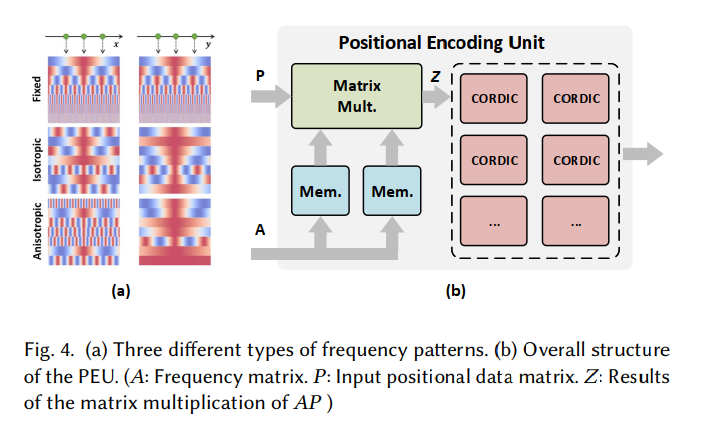### MLP Engine

MLP 引擎主要进行 $f(Wx+b)$ 类型的计算。

MLP 引擎包含有：

• 一个 Multi-output Network block (MONB)，负责计算中间的隐藏层
• 一个 Single-output network block (SONB)，负责计算最后的输出层
• 不继续用 MONB 的原因是，全连接的 MONB 比只输出一个数字的 SONB 面积要大得多
• 两个 activation memory block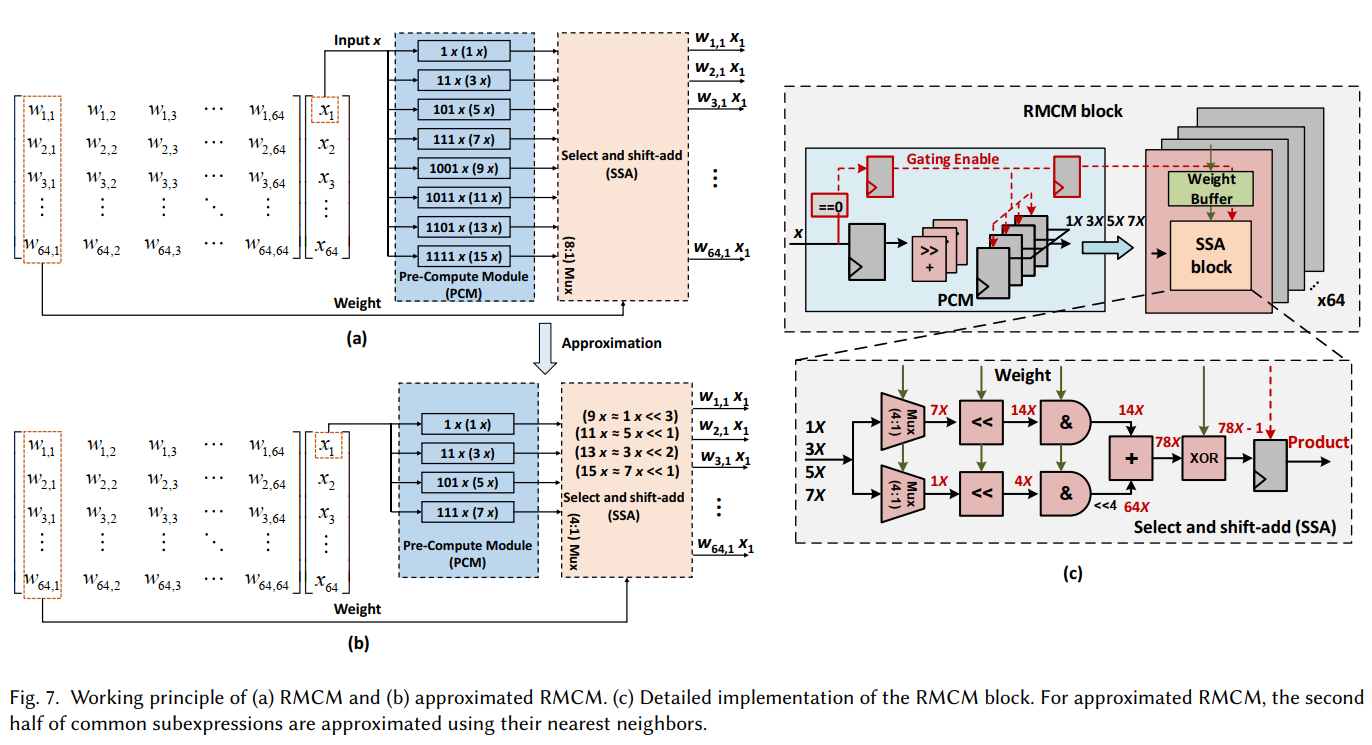$$3x = 1x << 1 + 1x$$

SONB 的架构基本上和 MONB 差不多，只是 RMCM 块用不到了，用普通的向量乘法块就可以了。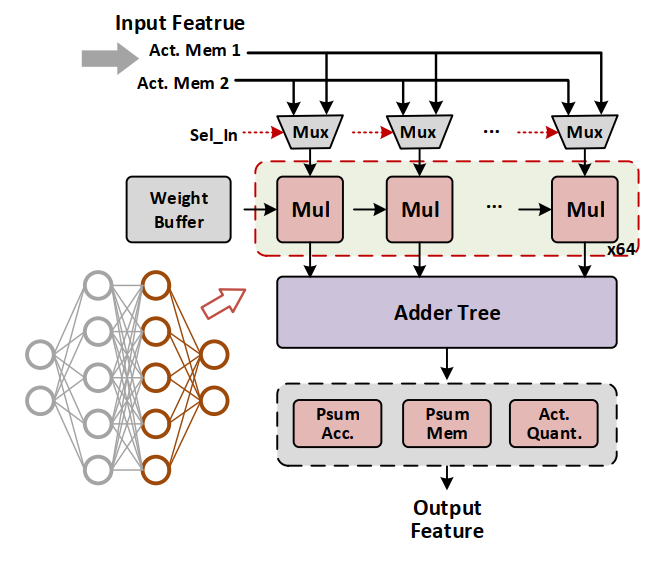### Volume Rendering Unit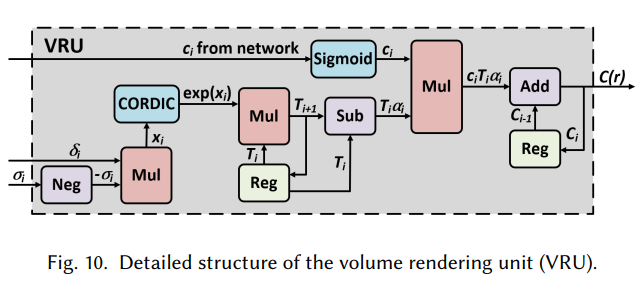VRU 模块主要要负责下面的计算：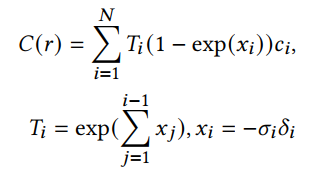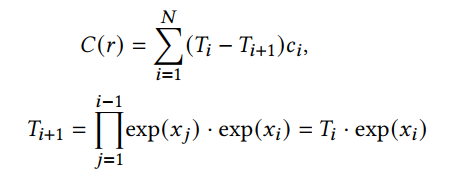### 原型验证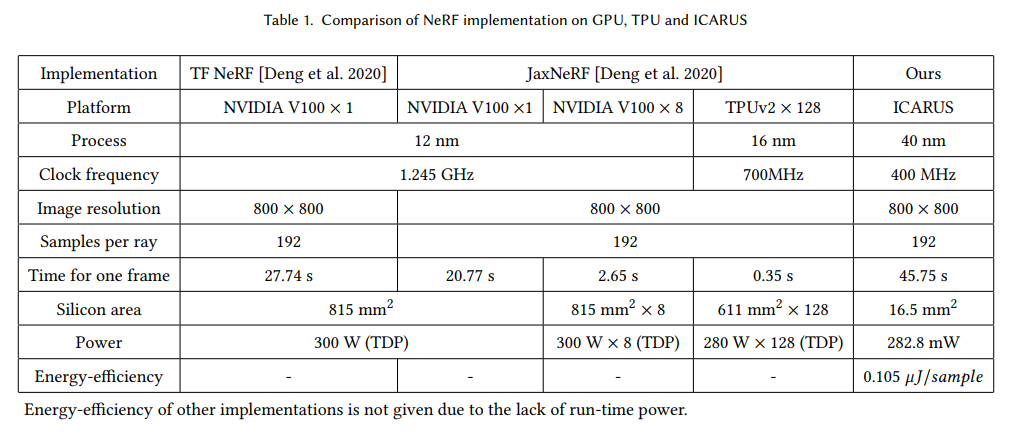## 简介

1. 从路径的角度出发，现有的特征线渲染方法将特征线处理成了光源
2. 特征线相交测试可以对任意的边开展，而不仅仅是在屏幕空间中

1. 对一整个路径中每条路径段分别进行和特征线的相交测试
2. 将交到的特征线视为吸收所有入射光，然后辐射用户自定义颜色的光源

TL;DR: 用 Path Tracing 做描边，把要描的边处理成光源，让描边也有景深、色散和反射等效果。

## 算法描述

1. 对每个 path segment 依次进行和特征线的相交判断，并且
2. 如果相交，则将特征线视为理想光源，并不再追下面的光源

### 相交判断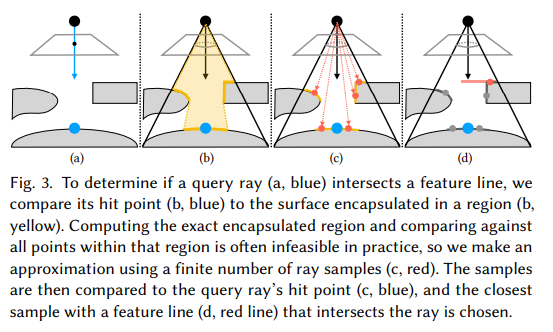### 采样权重修改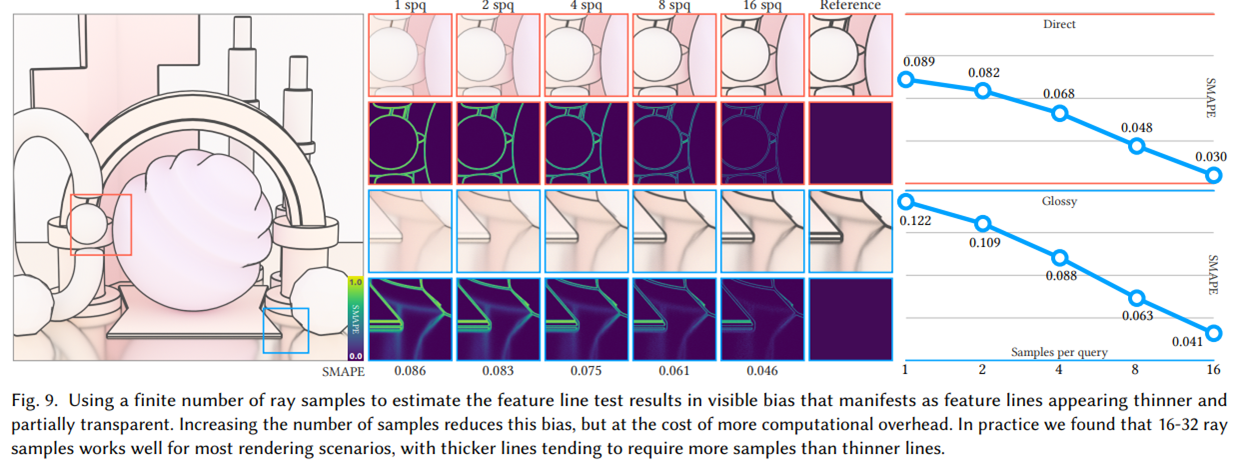### 特征线判据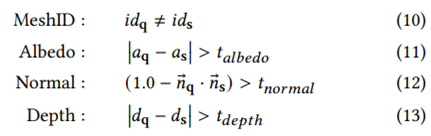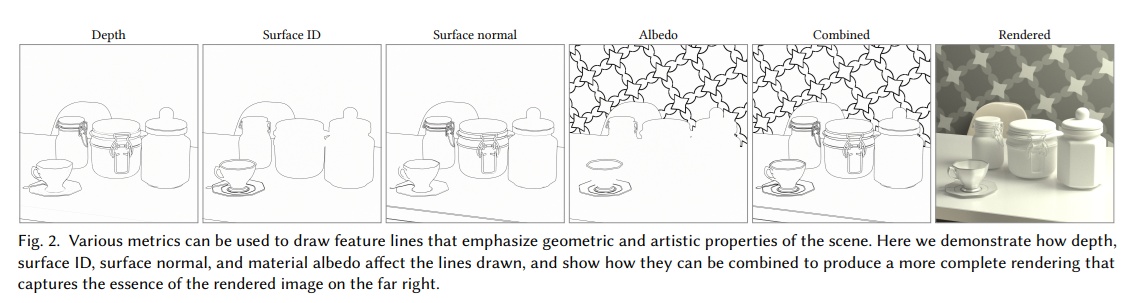## 效果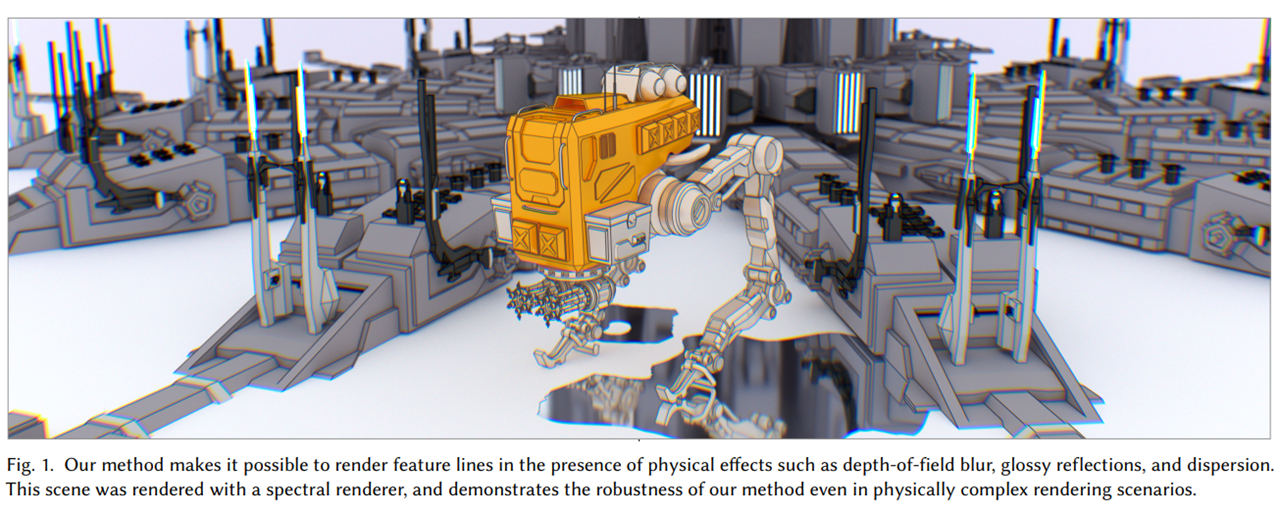## 未来的工作

• 其它路径采样方法 (i.e. BDPT)
• 特征线锥形区域估计改进
• 特征线区域缓存
• 特征线模型改进
• 反射 / 半透特征线模型等
• 将 lens blur 和色散效果集成到 Stylized Focus
• Stylized Focus 主要通过多个光栅化 pass 的叠加来实现风格化的景深和聚焦效果

Note: 大于号（>）小于号（<）目前没有做额外的转义（它们本身是 HTML 标签的结束和开始），但是这个用 \lt\gt 就行了，所以有点懒得改。

\begin{aligned} \nabla \cdot \mathbf{E} &= \frac {\rho} {\varepsilon_0} \\ \nabla \cdot \mathbf{B} &= 0 \\ \nabla \times \mathbf{E} &= -\frac{\partial \mathbf{B}} {\partial t} \\ \nabla \times \mathbf{B} &= \mu_0\left(\mathbf{J} + \varepsilon_0 \frac{\partial \mathbf{E}} {\partial t} \right) \end{aligned}

markedhexo-renderer-marked 使用的 Markdown 渲染器，它负责把 Markdown 渲染成 HTML。

## 为什么要新造一个轮子？

Google 一圈，现在有的 Hexo 内联 LaTeX 的方案都不是很让人满意：

1. hexo-renderer-kramed 使用的 kramed 从 2016 年开始已经没有再更新了
2. marked 表示不会加入对 $...$$$...$$ 的支持 (markedjs/marked, Issue #722)
3. hexo-renderer-pandoc 需要用户自己安装 pandocpandoc 本身很庞大，并且是 Haskell 编写，本文作者表示改不动；另外，直接 out-of-box 的装上之后，块公式 pandoc 总是会多生成 $...$ 的 pair，决定弃坑
4. 网络上还存在一些 patch 方案，比如这里，直接把 marked 的 inline rule 改掉，让其不再将 _ 作为合法的强调标志（比如 _asdf_ 之类就不会渲染成 asdf 了）
5. 其实也可以 摆大烂，把所有 LaTeX 和 Markdown 冲突的关键字都用反斜杠转义掉

## 改动简介

marked 采用正则表达式不断匹配的方式进行词法分析，对于部分块对象会继续进行行内的词法分析。词法分析后的 Toekn 流会送到 Renderer 进行输出。

## （作为菜鸡）踩过的坑

1. NodeJS 的 require 在找不到 index.js 时，会去 package.json 中查找 main 字段，并且加载对应的模块。

可以注意到，markedmain./lib/marked.cjs，这个文件需要运行 npm run build 生成。

2. 调试 Promise 链可以采用 Bluebird 的Promise.longStackTraces()

## 相关工作

math-marked这个项目在我基本写完之后才看到，不过作者没有基于原来的 commit 继续改，后续升版本会比较费劲。